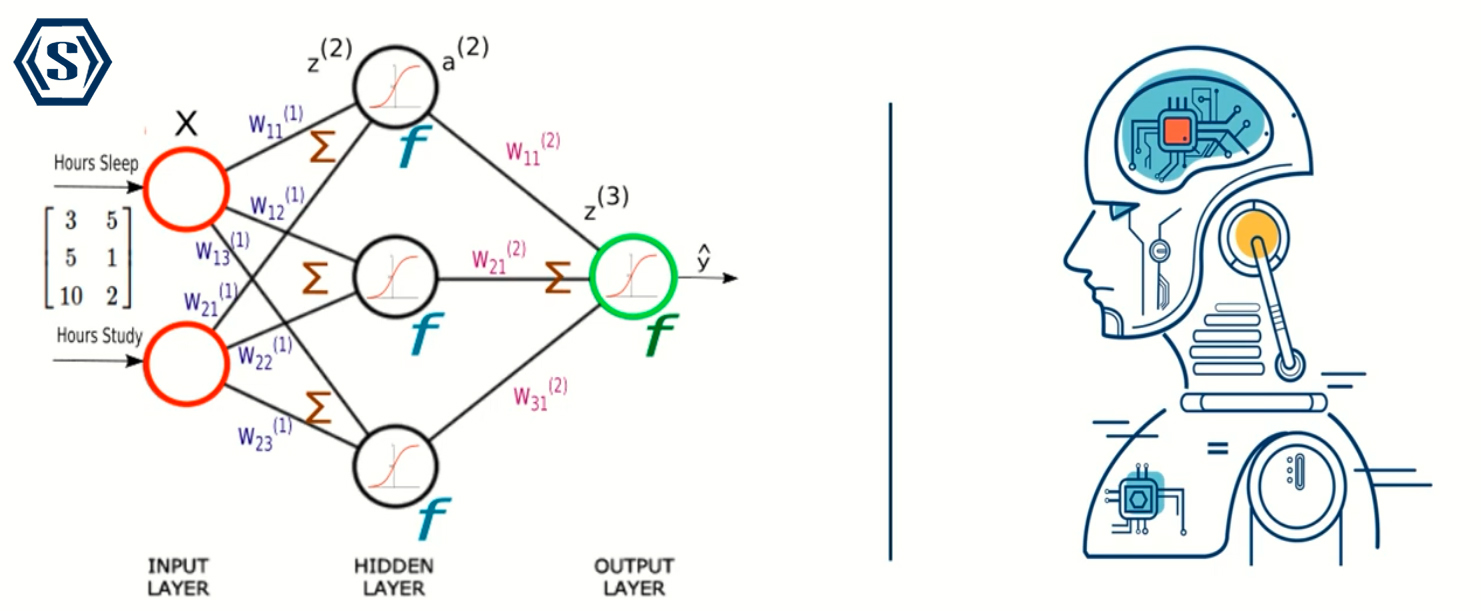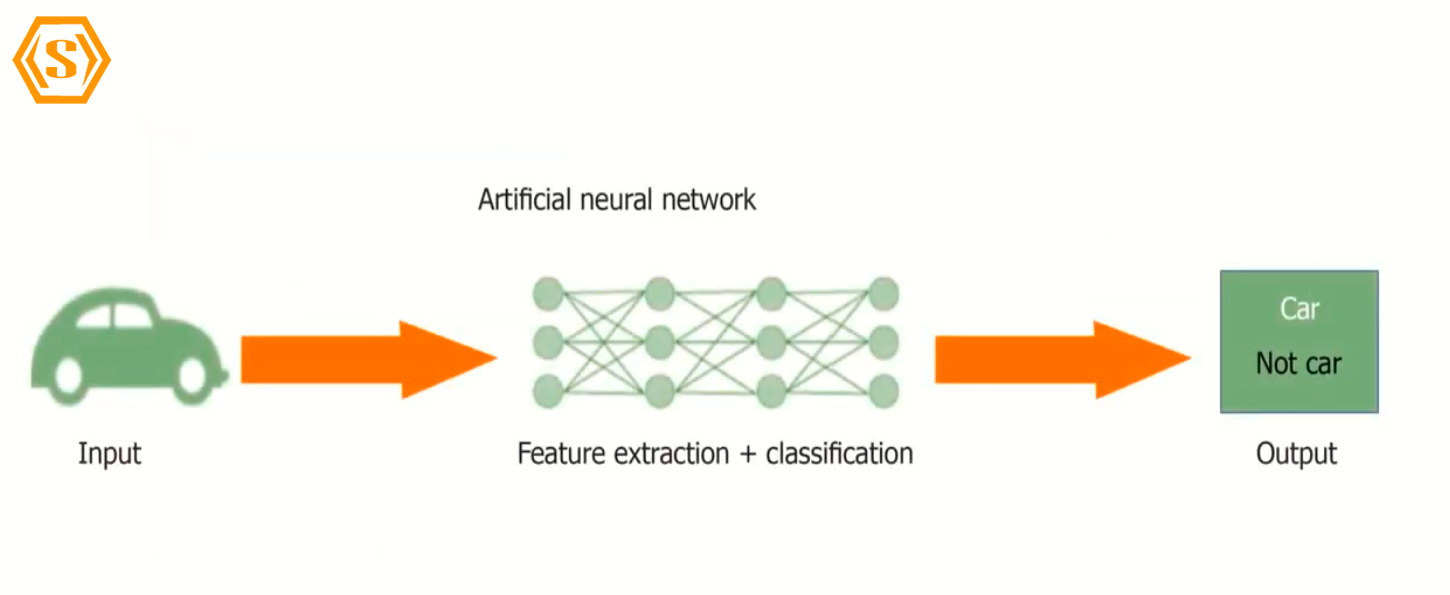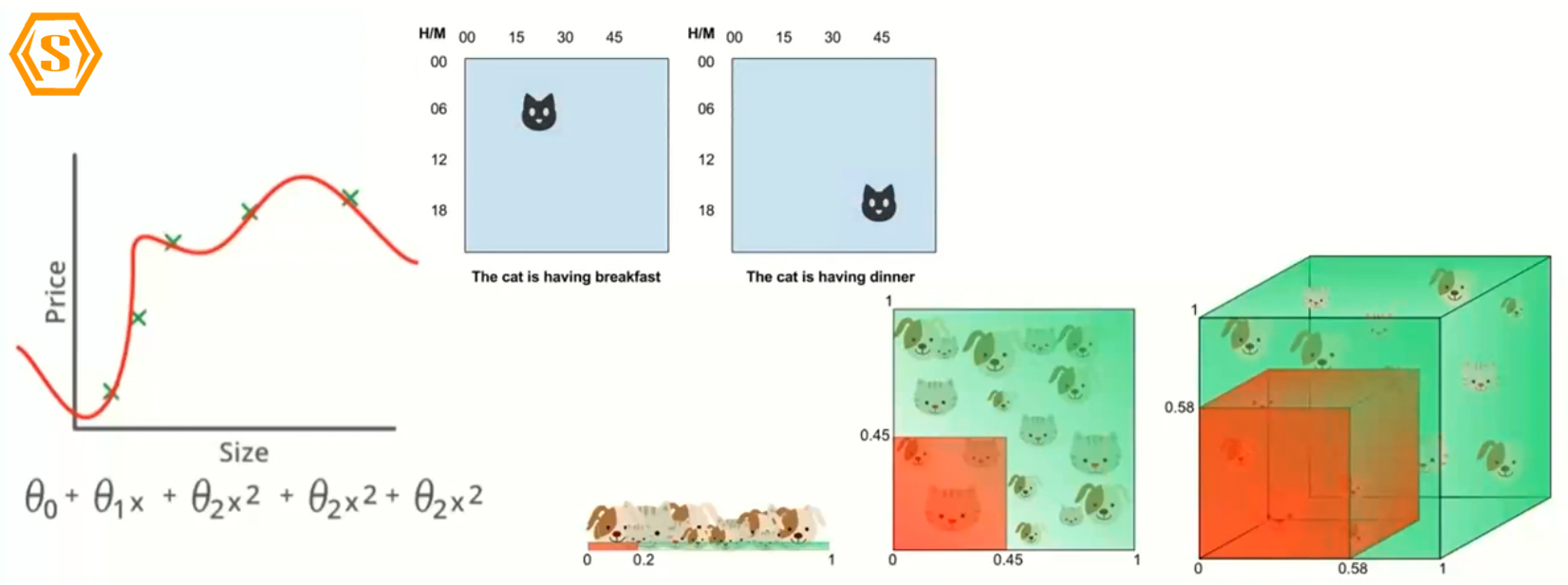Back to blog
Filter by:
Tags
Categories
//Article title

## Does Convolution Neural Network Algorithm can detect Covid?

01 Aug 2021Category :

Hi everyone, this is Shiroz here. Once again happy to share some knowledge with you guys. and I welcome you all to this interesting session where we'll be seeing how to detect Covid using chest x-ray so without any further ado let me quickly walk you through today's plan.

We'll start this topic by understanding the fundamental concepts of the algorithm that I used. Today we are going to cover several topics under That is CONVOLUTION NEURAL NETWORK.

• Why CNN?
• What is CNN?
• Image Processing Using CNN.
• CNN for Covid Detection.

Moving ahead we shall see how images are being processed using CNN or convolution neural network and finally, we'll end this topic by implementing CNN algorithm for detecting Covid and patience x-ray.

WHY CNN?so why CNN? Right, well before we come on to answer that, let's understand what are the basic components that go in here. CNN is an advanced version of an artificial neural network. now you might be wondering what is artificial neural network is right? well, an artificial neural network is a piece of a computing system is designed to simulate the way humans analyze and process information.

It is a foundation of artificial intelligence and solves problems that would prove impossible or difficult by human standards CNN have self-learning capabilities that enable them to produce better results as more data becomes available to them, you see what I'm trying to say here is, CNN has something called as neurons and each of these neurons are interconnected to each other with a link and let me tell you each of these neurons are capable of producing their own decisions.

The way this model tries to correct whatever the classification is done is by backpropagation. so now that we know what is CNN, let's see whether we can use CNN for images.Well if you are trying to ask me can I use CNN for images well the answer for this is simply YES.

Artificial neural networks can definitely, be used for images, here the way these works is whatever the image is there it will be converted in the form of a matrix, and we all know this matrix is nothing but pixel values, and when these pixel values are passed through an artificial neural network, it tries to analyze it and gives out kind of good results.

Now you might be wondering? if any is capable of image processing then why do we have a convolution neural network.Well, you see images are more than just pixel values, so images have features and patterns, so what I'm trying to say here is that, the artificial neural network fails to capture the pattern, so let me tell you like for example if as you can see an image of a cat over here, artificial neural network over here knows that this pixel value over here is black.  but it cannot see the pattern that is over here, and this is exactly why we use CNN. CNN or convolutional neural network is more or less about detecting how patterns are, and one of the reasons why we have to use CNN is because of three major reasons.

first, off we have something called as cursive dimensionality as we have a huge number of points in an image you know it becomes evitable over here, so this is one reason.  The second reason why we should use CNN is because of image variance as I mentioned earlier when we use ANN it's all about flattening the image and it tries to read an image pixel by pixel.

The next reason to use CNN is because of the overfitting problem, as you can see in this image, we have a huge amount of data set. and what this will do is our neural network will by hard each of these particular data points. and now when I fit a new image what happens is it will fade to recognize it and the reason for this is because our model is kind of fixated on learning only the similar kind of problems.

So there are three major reasons let me quickly give you a recap first one is the curse of dimensionality then we have an image variance and finally overfitting issues. Now that we know why we should use CNN.

WHAT IS CNN?Let us now quickly discuss what exactly is a convolution neural network, well the convolutional neural network is a deep learning algorithm which takes in images as input and assigns importance to various aspects or objects in the image, and it can create a differentiation between one image or one aspect from the other, the pre-processing required in convolution neural network is much lower as compared to other classification the algorithm.

The architecture of convolution neural network is similar to that connectivity pattern in the neurons of a human brain, and this was inspired by the visual cortex of a human being, here individual neurons respond to the stimuli only, so what I'm trying to say here is the neuron response only if that a specific feature is present, so this is what exactly is a convolution neural network.

So as you can see here we have image that is an input, and then we pass it through various filters, and each of these filters is responsible for taking one or the other feature and now once these features are formed we can reduce the size by using some meshes and then we perform the similar task again and again once we sample the image to a certain a level we flatten these images or I can say we flatten up these layers and then we pass an artificial neural network over it.so let me show you how exactly this works, as you can see here we have an image of a spacecraft and then there would be a slider which goes through all the pixel values and if if you say slider is of 3 crosses 3  then it takes three crosses three pixels at a time and goes through every pixel over here or the grid of pixels over here, and then we pass out a convolution and a relu which is an activation function, the reason why we do this multiple number of times is because we want to increase the non-linearity and basically over here we are trying to extract different features of an airplane.

Once we have enough number of features what we do is we flatten these features into a single strip of images, and then we pass them through a fully connected layer and out of which then we can classify whether an image is airplane or not and depending upon the type of the classification we can use the activation function, if the type of classification is binary we can use something called a sigmoid and if it's a multi-class classification we can use softmax.

Let us move ahead and see what are the basic components that go in when we are processing images using CNN.

CONVOLUTION LAYER

---------------------------------First off we have something called as convolution layer and what this convolution layer does is that it is a set of filters and this filters when passing through our image or our image data set it is capable of extracting a specific set of characteristics.

-------------------------

The next thing that we are going to use over here is going to be padding layer so usually what happens is when we pass a convolution layer the image size usually decreases by two pixels, the reason for this is because we are passing this particular image in that particular manner.we are passing this convolution filter now sometimes we don't want to lose a feature, sometimes a feature might be present at the corner right so what should I do if that's a scenario well that's when we have a padding layer the padding layer is basically adding a pixel whose value can be zero or one across this particular image and now what happens is the image after passing the size of the

image after passing it through a convolution filter would remain the same. So this is what is called as padding layer or adding paddings to our images.

MAX POOL LAYER

--------------------------In max pool layer we won't be learning anything new it's basically used to reduce the

dimensionalities over here and the way max pool layer works is that you know we can pass out a 3 cross 3 grid or filter and what this would do is this would just take in the maximum value in that particular grid.

So if you can see over here there is this filter right max filter and this goes through three crosses three grid over here and whichever is a the maximum value in this three cross three

that particular value is given out and if you are asking me why am I using maximum value over here, the reason is that if I say in this particular matrix.let's say 7 3 8 7 over here 8 is a maximum the value so this means that 8 is contributing maximum

or the highest to that particular image or that particular feature in that image so that's why I'm taking out the maximum number in this matrix grid.

This is what exactly is max pool layer and this is what it does, so now that we know what is the convolutional neural network let me now quickly show to my code editor and show you how we can use CNN for detecting covered in our x-rays all rightSo as you can see the code editor that I'm going to use today is google collab. So now I have my data set which is available here which I've already loaded so I'll be creating will have this training data set which has both covered and normal and then I'll also have this validation data set for both covered and normal so let me quickly show you one or two images over here.So these is images for covid. so as you can see here, you know you can see the x-ray is kind of foggy and we have a couple of patches over here and now if I have to show you the normal image.So this is the normal image of an x-ray so as you can see here, we obviously don't have much detail information or we personally can't analyze any features over here that's because we obviously aren't radiologists or doctors,

So what we're going to do here is we'll be feeding this label data to our deep learning algorithm and then we'll see whether our algorithm works fine or not.So let me quickly zoom in over, so now what I did, import a couple of libraries that we need, so I have import numpy as np then we have we obviously need uh some matplotlib import matplotlib as pipelord let's import some deep learning layers so from keras dot layers import dense layer then we need a convolution layer so it would be convolution 2d and then we have max pool layer all right and then we obviously need a dropout so that we can prevent overfitting and then finally we have to pass our whatever the outputs of the CNN we have we have to pass it through our n right so for that we have to flatten our layer so that's why we are going to use flatten than we have from models that are Keras dot models import uh here we can go with one of two ways we can use the functional API or sequential model so I’ll be using sequential and then I'll also import

from Keras because we have to load our images right so I don't want to use OpenCV I can just use the image library which is present over here so Keras are pre-processing import images and just import Keras.After some are the progress. Build a CNN model first, off we'll have to initialize the sequential so let's say the model is equal to sequential, so added a couple of layers so first off we have to add convolution layer so model dot add and then over here we'll say contour d, and here we have a number of filters if you remember as I mentioned earlier number of filters represents the features that you would extract and usually when you are in the starting,  you will be using less number of

filters this because this usually takes lower level features and lower level features aren't that important because we won't be getting much information from lower level features then we have kernel size that is the size of a filter usually it's three crosses three and the reason it's three crosses three is because it has become an industry-standard after the Alex net, and then we obviously have to involve uh non-linearity,

So it 'll be using relu and finally, you will need input shape and the input shape usually has to be

same as what we're using here so it's going to be 256 comma 256 comma 3 3 is because it's an RGB image so it's going to be 3 channels right now we are done with the first convolution layer.so as you can see here in the first layer, we have 896 parameters, and yeah as we are assing through the first one so we have 254 the size of the image is reduced here as you can see, and as we go down the number of parameters are increasing and if you can see here max pool 2d and dropout we are not learning any new parameters.When i finally, flatten this we have this many number of parameters that is almost around 73 lakhs, so yeah we have 73 lakh parameters which is fine and now we are going to finally, train our model so to train our model.So as you can see here our training is successfully completed and our model has performed quite good. as you can see here our accuracy has obviously increased and our validation accuracy at one point has become hundred percent I can say that it has overfitted some of this model and we can actually enhance our learning by you know by having hyperparameter tuning and we can also do some early stopping techniques so with that the way we can get an optimal accuracy on our validation data.So  we know that this is a covid image and now our model should predict whether the image is covid or not so it's giving me this class 0.So let's see what does class 0 represents here as you can see here class 0 represents images covid.Now let's take normal image here all of these images are nothing but the covered x-rays,let’s take a normal image. It gave me class one so it's working pretty great. if I pass out any of the images it's working totally great.

All right so this is there that is all about detecting covid using x-rays alright guys with this we come to the end of our today topic. I hope you have enjoyed reading to this artical please be kind enough to like it and you can comment any of your doubts and queries and I will reply them.